RUSSIAN JOURNAL OF EARTH SCIENCES, VOL. 13, ES1002, doi:10.2205/2013ES000527, 2013

# Modulating impact of electromagnetic radiation on geoacoustic emission of rocks

V. A. Gavrilov1, I. A. Panteleev2, G. V. Ryabinin3, Yu. V. Morozova1

1Institute of Volcanology and Seismology, FEB RAS, Petropavlovsk-Kamchatsky, Russia

2Institute of Continuous Media Mechanics, UrB RAS, Perm, Russia

3Kamchatka Branch of Geophysical Survey of RAS, Petropavlovsk-Kamchatsky, Russia

.

### Abstract

This work presents the results of studies of a physical basis of modulating effects of continuous exposure to electromagnetic radiation on intensity of geoacoustic processes in real geological media. The study is based on data from long-term borehole geoacoustic, electromagnetic, hydrogeochemical and hydrogeodynamic measurements performed in the Petropavlovsk-Kamchatsky geodynamic polygon. The authors have analyzed the physical reasons for the high correlation of the RMS signals of geoacoustic emission and external electromagnetic radiation at intervals of background seismicity and investigated the physical mechanism of the effect of degradation of geoacoustic emission response to the electromagnetic effects observed at the time of relatively close strong earthquakes.

### Introduction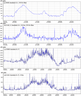Figure 1

The earlier results of long-term borehole geoacoustic and electromagnetic measurements carried out in the Petropavlovsk-Kamchatsky geodynamic polygon, have shown the effect of modulating electromagnetic impact on intensity of geoacoustic processes [Gavrilov, 2007; Gavrilov and Vlasov, 2011; Gavrilov et al., 2006, 2008, 2011]. The essence of the effect is that for seismically quiet periods the envelope of geoacoustic emission (GAE) signal at measurements in boreholes has a high degree of correlation with the envelope of intensity signal of the electric component of external electromagnetic radiation (EMR) in the area of the same borehole. As an example Figure 1 shows the results of geoacoustic and electromagnetic measurements in the Boreholes G-1 and K-33, located at a distance of 36 km from each other. The data of measurements are presented in the form of root mean square (RMS) values. The RMS graphs of EMR and GAE for the Borehole K-33 were obtained after a digital filtration of initial data by a top frequencies filter with a 150 Hz cutoff frequency in order to eliminate the traffic-related noise from a road, located at a distance of 350 m from the borehole. The data in Figure 1 show that for seismically quiet periods RMS variations of geoacoustic emission are actual responses to external EMR.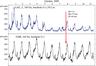Figure 2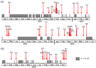Figure 3

Time neighborhood of strong ($M_{LH} \geq 5.0$) close earthquakes complicates the effect of modulating electromagnetic impact. As a rule, in these cases we observe a degradation of GAE response to EMR (Figure 2). Results of the analysis of long-term series of measurements show that the effect of degradation of GAE responses to EMR is rather stable as an indicator of stress-strain state of geological media, at least for geodynamic processes of regional scale. Figure 3a and Figure 3b present the results of comparison to seismic activity of the geoacoustic data obtained at measurements in Borehole G-1 in 2001–2003 (Figure 3a) and in 2010 (Figure 3b). For the data presented in Figure 3a, an excess of daily variations amplitude $a$ over a threshold value $a_{th}=500$ RU at a maximum value of amplitude $a_{\max}=2804$ RU served as a criterion of existence of GAE response. At data processing for 2010 (Figure 3b) a criterion of existence of GAE response to EMR was an excess of threshold value $\rho = 0.35$, for correlation coefficient $\rho$ between EMR and GAE variations in a daily window.

The data in Figure 3a show that EMR degradation took place before all 37 earthquakes with $M_{LH} \geq 5.0$ in a zone of $R \leq 300$ km from a borehole and the earthquakes with $M_{LH} \geq 5.5$ in a zone of $R\leq 550$ km. The probability of a casual coincidence of such situation is estimated to be less than $10^{-6}$ (seismic data correspond to the catalog of earthquakes of the Kamchatka branch of the Geophysical Survey of RAS).

The applied character of the specified results stimulated the works directed on research of the physical reasons and mechanisms of specified effects as bases for development of new methods of complex geophysical monitoring of a stress-strain state of geo-environment and for predicting earthquakes. Among the latest works of this direction, we would like to mention [Ryabinin et al., 2011, 2012] which draws attention to existence of correlation between the changes of amplitude of daily variations of GAE in Borehole G-1 and changes of water mineralization in this borehole before a swarm of earthquakes occurring in Avacha Bay (Kamchatka) in October, 2001.

Results of the detailed research in which the main objective was the study of a physical basis of effects of EMR on intensity of geoacoustic processes in real geological media are presented in this article. Researches were conducted on the basis of the long-term borehole geoacoustic and electromagnetic measurements which are carried out by the Institute of Volcanology and Seismology of Far Eastern Branch of the Russian Academy of Sciences (IVS FEB RAS) in the Petropavlovsk-Kamchatsky geodynamic polygon, and also on the hydrogeochemical and hydrogeodynamic observations made in the same territory by the Kamchatka Branch of the Geophysical Survey of the Russian Academy of Sciences.

### Technology and Method of Observations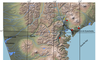Figure 4

Borehole geoacoustic and electromagnetic measurements are carried out in the Petropavlovsk-Kamchatsky geodynamic polygon from August 2000. In November 2012 the network of observations consisted of four radio telemetric points, set up on a basis of Boreholes G-1, R-2, E-1, K-33, and the Center for collecting and processing data, located in the building of IVS FEB RAS (Figure 4). The measurements focused on monitoring of a stress-strain state of geological media and seismic hazard prediction. Geoacoustic and electromagnetic measurements are carried out at all network measuring points. At some points they are supplemented by other types of measurements. Figure 4 also shows the location of Borehole GK-1, used for hydrogeochemical and hydrogeodynamic observations.

#### Geoacoustic Measurements

So far the main volume of geoacoustic and electromagnetic data was obtained by measurements in Borehole G-1, where research was carried out since 2000. Till September, 2009 researchers made measurements using a geophone with sensors on the basis of crystal ferromagnetics. A signal at the exit of a sensor of this kind is proportional to the third derivative of soil shift, and the steepness of amplitude-frequency characteristic (AFC) of the sensor in recalculation to soil shift makes 60 dB for a decade of frequency variation [Belyakov, 2000]. The geophone was installed at a depth of 1035 m. From September 2009 geophones with piezoceramic A1612 sensors manufactured by "Geoakustika" Co. were used, as a rule, for borehole geoacoustic measurements. Working range of frequencies of sensors makes $0.1 \div 1000$ of Hz on level 0.7. Signal at a sensor's exit is proportional to acceleration, respectively the steepness of AFC in a working band of frequencies makes 40 dB for a decade of frequency variation. Continuous geoacoustic measurements in Borehole G-1 are carried out using A1612 sensors at the same time at two depths: at a depth of 1012 m with a use of a three-component geophone and at a depth of 270 m where a unicomponent geophone was installed.

In some cases analog and digital hydrophones are used for borehole geoacoustic measurements. In particular, researchers use an analog G61N hydrophone, produced by "Geoakustika" Co., for continuous geoacoustic measurements in Borehole K-33 (see (Figure 1d).

There are two modes of borehole geoacoustic and electromagnetic measurements: a monitoring mode and a mode of experimental measurements.

Monitoring mode is used for continuous measurements of RMS values of GAE and EMR signals in a range of frequencies of 25–1400 Hz. In this mode of measurements initial broadband signals arriving from exits of a geophone or underground electric antenna, are exposed in the beginning to analog processing: strengthening, filtration, two and a half period rectification. Then a digital averaging of signals in a given time interval which can change remotely from 1 to the 18th hour (1 min on default) follows. Such scheme of processing of incoming signals allows to reduce the volume of data of geoacoustic and electromagnetic measurements registered and transferred by communication channels. During the measurements in Borehole G-1 GAE and EMR signals were filtered by one-third octave band pass filter with central frequencies of 30, 160, 560 and 1200 Hz. Regular value of an interval of averaging was 1 min. The measuring complex is described in detail in [Vlasov et al., 2008].

Experimental measurements mode was applied to high-precision registration of wave forms of initial signals of GAE and EMR in frequencies ranging from 5 Hz to 10 kHz for research of spectral and time structures of GAE and EMR. For carrying out such measurements we used a digital Zet220 module with a 24-digit ADT and with a maximum frequency of transformation of 8 kHz, produced by "Elektronnye tekhnologii i metrologicheskiye sistemy" Co. Processing of data, obtained with a use of Zet220 module, was generally carried out by WinPOS software package (developed by R&D enterprise "Mera").

#### Electromagnetic Measurements

According to the problem-solving tasks the data of electromagnetic measurements were used in two applications. First, they served as a basic signal for rationing of GAE response to EMR. Secondly, the data was used for evaluating changes of conductivity of rocks around a borehole. Electromagnetic measurements at all measuring points of Petropavlovsk-Kamchatsky polygon (see Figure 4) were carried out with underground electric antennas. Location of a borehole (an urban area, a forest area, etc.), EMR level around it and a borehole's configuration determined the choice of a concrete type of antenna. Measurements in Borehole G-1 were carried out with an underground electric antenna. Basic components of this antenna were a bypass tube of the borehole casing and a sealed armored cable about 1000 m long, lowered in the borehole [Gavrilov et al., 2006]. According to this antenna's configuration we considered the bypass tube as a linear element with a length of $h$ of an asymmetrical vibrator (a monopole), and the cable cord inside the bypass tube as an element of inductive communication of the antenna with entrance intensifying cascade. Assuming that conductivity of the top layers of soil is much higher than conductivity of deeper layers, at the expense of mirror display of such a monopole this antenna can be presented in the form of a dipole with a length of 2 h. Use of a bypass tube as a basic element of the antenna uninsulated from geological media results in strong dependence of level of signals at the antenna exit on electrophysical parameters of the surrounding geo-environment. That fact allows us to use such antenna for monitoring changes of conductivity of rocks surrounding a borehole. Therefore electrophysical parameters of nearby rocks will have an impact both on characteristics of the antenna and the EMR level at its entrance.

Dependence of conductivity at the antenna entrance on geological parameters is determined by the coefficient $a$ of EMR damping in semiconducting media [Krasjuk and Dymovich, 1974]:

\begin{eqnarray*} \alpha=\omega \sqrt{0.5 \mu_a \varepsilon_a(\sqrt{1+\sigma^2(\omega\varepsilon_a)^2} -1)} \end{eqnarray*}

where $\sigma$ – specific conductivity, $\varepsilon_a$ – absolute dielectric permittivity of geological media, $\mu_a$ – absolute magnetic capacity of geological media.

Regarding changes of characteristics of the antenna, as it was shown in [King and Smith, 1981], first of all, entrance impedance of uninsulated $Z_{en}$ antenna significantly depends on surrounding media parameters. For frequencies, at which the electric length of the antenna is close to the resonant length, $Z_{en} \sim \varepsilon^{1/2}$ (such situation, in particular, takes place at frequencies about 160 Hz for the antenna of Borehole G-1, where a 2542 m long bypass tube is used as a linear element). In such case changes of specific conductivity and dielectric permeability of geological media, related to changes of its fluid saturation capacity, will have unidirectional effect both on antenna characteristics, and the EMR level at its entrance.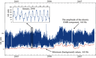Figure 5

To evaluate changes of conductivity of the rocks surrounding the borehole, special processing of RMS series of EMR with separation of background EMR was carried out (Figure 5). Processing provides smoothing of daily variations of RMS values of EMR and calculation of the minimum values of a series in a sliding window about 20 days wide. For the first time the specified technique was used for data processing of electromagnetic measurements in time vicinity of the catastrophic Simushir earthquakes of 2006–2007 [Gavrilov, 2009].

#### Hydrogeochemical and Hydrogeodynamic Measurements

Special observations of underground water regime in Kamchatka, focusing on identification and research of hydrogeochemical anomalies connected with preparation and realization of earthquakes, have begun in 1977. Regime observations provided measurements of outflow of water and temperature of self-streaming boreholes and sources, water and gas sampling for the further analysis in vitro, measurements of atmospheric pressure and air temperature. Water tests have defined water pH, concentration of ions of chlorine (Cl$^-$), hydrocarbonate (HCO$_3^-$), sulfate (SO$_4^{2-}$), sodium (Na$^+$), potassium (K$^+$), calcium (Ca$^{2+}$), magnesium (Mg$^{2+}$), concentration of boric (H$_3$BO$_3$) and silicon (H$_4$SiO$_4$) acids. The tests of gas dissolved in water have defined methane (CH$_4$), nitrogen (N$_2$), oxygen (O$_2$), carbon dioxide (CO$_2$), helium (He), hydrogen (H$_2$), hydrocarbonic gases: ethane (C$_2$H$_6$), ethylene (C$_2$H$_4$), propane (C$_3$H$_8$), propylene (C$_3$H$_6$), butane (C$_4$H$_{10}$n) and isobutane (C$_4$H$_{10}$i). The mineralization was calculated as the sum of concentration of the main indicators of cationic and anionic components of water (HCO$_3^-$, Cl$^-$, Ca$^{2+}$, SO$_4^{2-}$, Na$^+$, K$^+$, Mg$^{2+}$).

This work used the results of measurements of water mineralization of Boreholes G-1 and GK-1, as borehole as of the water outflow of GK-1. The boreholes are located in the territory of Petropavlovsk-Kamchatsky geodynamic polygon at a distance of 35 km from each other (Figure 4). Water comes out from both boreholes, but they differ considerably in amount of water outflow on surface. Average annual outflow of GK-1 was about 0.1 l/s, at the same time for G-1 this figure was about 0.00003 l/s. The measurement resolution for Boreholes G-1 and GK-1 was three days. The water of G-1 contains mainly chlorine-ion, with average annual value of its concentration about 6600 mg/l and sodium-ion (4200 mg/l). The ionic composition of water of Borehole GK-1 was predominantly chloride (5500 mg/l) and sodium (2500 mg/l). Relative random error of water mineralization of G-1 and GK-1, representing a sum of random errors of measurements of cationic-anionic concentrations, was about 1.0%. Results of the study of hydrogeochemical effects, accompanying the Kamchatka earthquakes, can be found in [Bella et al., 1998; Biagi et al., 2000, 2006; Khatkevich and Ryabinin, 2006; Kopylova et al., 1994; Ryabinin and Khatkevich, 2009].

Concerning these hydrogeochemical measurements we would like to mention the following. According to the estimates made in [Gavrilov, 2007], the main contribution to the results of borehole geoacoustic measurements was made by the processes occurring in the geological media within a radius of tens – first hundred meters from a point of installation of a geophone. In this regard for research of influence of the fluid content of rocks on intensity of geoacoustic processes the data of geoacoustic measurements in Borehole G-1 were compared to data of measurements of water mineralization for the same borehole. At that the questions concerning the relation of registered changes in mineralization of water of boreholes to parameters of the centers of earthquakes and hydrogeological conditions weren't considered.

The data of measurements of outflow from Borehole GK-1 were used for estimating the character and levels of the influencing tectonic tensions. It is known that changes of water outflow of boreholes in some cases reflect changes of volume deformations in geological media [Asada et al., 1984; Sidorin, 1992]. The correct use of such data, as a rule, requires compensation of seasonal variations. In this case such procedure wasn't applied as the analysis of long-term (about 15 years) series of measurements of outflow of GK-1 showed a lack of seasonal variations. Such result can be explained by the fact that Borehole GK-1 drains generally deep thermal waters of a zone of the complicated water exchange, and their regime is poorly subject to influence of seasonal changes of groundwater conditions. Taking all this into account, we interpreted the results of complex borehole measurements assuming that the data on changes of the outflow of GK-1 reflected the changes of volume deformations and tensions, influencing the geological media.

### Main Results of Measurements and Their Discussion

#### Correlation of Amplitudes of GAE Response and Values of Water Mineralization in the Boreholes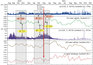Figure 6

As a whole the results of research have confirmed the conclusions drawn in [Ryabinin et al., 2011, 2012], concerning the existence of correlation between the changes of amplitude of GAE response and water mineralization in Borehole G-1. At the same time, the detailed data analysis showed that the nature of this correlation is not insighificant due to the technique of hydrogeochemical measurements and specific features of the borehole's design. As a corresponding example Figure 6 shows the results of measurements of mineralization of water of Borehole G-1 (Figure 6b) in comparison with simultaneous geoacoustic measurements in G-1 at depths of 1012 m (Figure 6c) and 270 m (Figure 6a). The chosen interval of measurements (August, 2010–June, 2011) is interesting because it includes the major seismic events, for which the value $S=L_P/R_h \geq 3.3\%$ ($L_P =10^{0.44M-1.29}$ – seismic center length [Riznichenko, 1976]; $R_h$ – hypocentral distance). On the specified time interval by criterion of $S=L_P/R_h$ a megaearthquake ($M=9.0$) which has occurred on March 11, 2011 in Japan (the Tohoku area) at the epicenter distance of $R=2049$ km was the strongest event ($S=21\%$).

Data in Figure 6 show that the character of correlation between the amplitudes of GAE response and water mineralization of G-1 has changed with the growth of compressive stress (Figure 6e), beginning at the end of December, 2010. Before that changes in water mineralization in G-1 on the August–December, 2010 interval reliably correlated with changes of the envelope of amplitudes of GAE daily variations at a depth of 270 m (correlation coefficient $\rho \approx-0.9$), while for the same interval changes of amplitudes of GAE at a depth of 1012 m practically weren't connected with the changes in water mineralization of Borehole G-1 and the corresponding coefficient of correlation was close to zero. The nature of the specified correlation has essentially changed in the time vicinity of the megaearthquake in the Tohoku area. On the January–April 2011 interval the changes in water mineralization in G-1 began to correlate with changes of amplitudes of daily variations of GAE at a depth of 1012 m (correlation coefficient $\rho \approx-0.9$), and correlation with GAE changes at a depth of 270 m has practically ceased to exist.

Thus the data shown in Figure 6 allowed us to come to two conclusions. Firstly, in time vicinities of rather strong seismic events, high correlation of changes of amplitudes of responses of GAE at a depth of about 1000 m with changes in water mineralization of Borehole G-1 takes place. Secondly, on such intervals high correlation of variations of water mineralization of Boreholes G-1 and GK-1 was noted.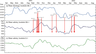Figure 7

It should be mentioned that according to the results of 11-year interval of measurements (2000–2011) high correlation of changes of water mineralization of Boreholes G-1 and GK-1 was noted in some other cases, initially, for time vicinities of rather strong seismic events. For example, we can point out at the results of measurements in a time vicinity of a swarm of strong earthquakes which took place in October, 2001 around Avacha Bay (Kamchatka) at the epicenter distance of about 130 km from G-1 (Figure 7). The strongest earthquake of the swarm ($Ml=6.3$) occurred in Petropavlovsk-Kamchatsky up to magnitude 5 accompanied by numerous aftershocks. As it is borehole illustrated by Figure 7, on the July 2001–March 2002 interval the changes in water mineralization in Boreholes G-1 and GK-1, located at a distance of 35 km from each other, occurred almost synchronously. The correlation coefficient between the series was $\rho \approx + 0.9$. Changes of amplitudes of GAE responses for a depth of about 1000 m in Borehole G-1 were connected with changes in mineralization of water at the borehole head. At that the corresponding coefficients of correlation had the values of the $\rho \approx-0.7$ order [Ryabinin et al., 2012].

It would be logical to assume that the increase in correlation of variations of water mineralization of Boreholes G-1 and GK-1 in time vicinities of rather strong seismic events is connected with growth of compressive tension on such time intervals. At the same time, taking into account very small outflow from Borehole G-1 the growth of compressive stress cannot explain the high correlation and more so the synchronism of variations of water mineralization of Boreholes G-1 and GK-1 in time vicinities of rather strong seismic events.

As we mentioned earlier, the water of GK-1 arrives from water-bearing horizons in the range of depths of 613–1261 m. Taking into account the values of self-outflow (0.1 l/s) and diameter of a borehole-casing tube the maximum time of water outflow from specified depths on a surface for Borehole GK-1 will not exceed two days. Water in Borehole G-1 flows through perforations in the borehole casing tube, located in the range of depths of 1710–2424 m. Taking into account the value of self-outflow on a surface (about 0.00003 l/s) formally calculated time of water outflow on the surface will not be less than 10 years, it obviously contradicts the results of measurements on intervals where almost synchronous significant changes of water mineralization at the borehole head of G-1 and of the water of the self-streaming Borehole GK-1 take place. On such intervals the water mineralization at the borehole head of G-1, as well as the water mineralization of GK-1 depends on the mineralization of deep water arriving on the surface with a delay of not more than several days.

The explanation of the obtained results can be related to the configuration of Borehole G-1. After the end of drilling in June, 1988 the outflow of water from G-1 was about 0.3 l/s. The outflow gradually decreased and in September, 1998 did not exceed 0.00015 l/s. It is possible to assume that the actual outflow of G-1 up to the present time remained rather high – about 0.3 l/s (as it was at an initial stage after drilling) – but because of violations of integrity of the borehole-casing column the main outflow of Borehole G-1 later began to happen in loose sediments below a day-surface. At that, if the main outflow of the borehole takes place at a low depth, then in periods of relatively strong tectonic tensions, when the outflow increases, water mineralization at the head of Borehole G-1 will depend on deep water mineralization. The assumption of the violation of integrity of the borehole-casing column of G-1 is indirectly confirmed by the report about the results of the borehole's drilling describing the accident which happened in the course of drilling – a break of four borehole-casing tubes at a depth of 260 m [Report..., 1988]. It is possible that a concrete bridge built to liquidate the accident in the range of 250–325 m in the course of time ceased to provide the necessary water-tightness of borehole-casing column, and the main outflow of the borehole began to happen below a day-surface.

It is necessary to emphasize that irrespective of the concrete reasons causing the specified nature of changes in water mineralization at the head of G-1, it is possible to make a reasonable conclusion that in time vicinities of relatively strong seismic events a high correlation of changes of amplitudes of GAE responses, registered in Borehole G-1 at a depth of about 1000 m, with changes in water mineralization at the head of the borehole takes place.

### Physical Mechanism of Effects of Influence of Electromagnetic Radiation on Intensity of Geoacoustic Processes

Based on modern ideas about a structure of real geological media, emphasizing such features as its granularity (blockiness) [Goldin, 2004], fluid saturation and heterogeneity of pore/fracture space [Kissin, 2009], we will consider the most real, in our opinion, physical mechanism causing effects of influence of external EMR on intensity of geoacoustic processes. Let us assume, as it is usually done in most cases, that geological media consists of low permeable blocks divided by more permeable channels (a system with double porosity).

Generally geoacoustic emission at depths of installation of geophones (200–1000 m) is connected with a noise of processes of repacking, fragmentation, compaction and cracking of fluid-saturated geological media under the influence of tectonic tensions, lithostatic pressure, seismic waves and flux flows. Existence of an ion-conductive fluid in pore/fracture space of geological media causes emergence of double electric layers (DEL) on borders between a fluid and a mineral skeleton of rock. Density of DEL charges at each such contact has an essential total impact on GAE level. With increase in density of DEL charges a friction coefficient for inter-phase borders also grows (it is indirectly confirmed by experimentally found change of parameters of friction under the influence of electric current [Chelidze et al., 2004]), that leads to breaking of processes of repacking, fragmentation and cracking and causes reduction of GAE level. With a growth of density of DEL charges a value of critical coefficient of intensity of tension for each of cracks also increases [Finkel, 1977], defining critical level of tension necessary to start the growth of a crack. It in its turn also leads to GAE level reduction. Change of sizes of DEL charges on the borders of fluid and solid phases in fluid-saturated geological media is defined by density of currents transferring charges in an ion-containing fluid (electrolyte). Generally in the presence of external electric field and a gradient of pore pressure a density of total current $\boldsymbol{j}$ in fluid-saturated media will be the sum of three vector variables: density of conduction current $\boldsymbol{j}_E$, density of diffusive current $\boldsymbol{j}_D$ and density of current of electro-kinetic nature $\boldsymbol{j}_{ek}$:

\begin{equation} \tag*{(1)}\label{1} \boldsymbol{j} = \boldsymbol{j}_E + \boldsymbol{j}_D + \boldsymbol{j}_{ek} \end{equation}

Let us treat the fluid filling the geological media as binary electrolyte. Competency of such assumption is confirmed by results of measurements of ionic composition of water in Boreholes G-1 and GK-1 showing that the selected tests contain mainly chlorine-ions and sodium-ions.

In the presence of external electric field with intensity density of conduction current in the media with specific conductivity $\sigma$ is:

\begin{eqnarray*} \boldsymbol{j}_E = \sigma \boldsymbol{E} \end{eqnarray*}

Density of diffusive current for the case of binary electrolyte can be presented as [Svetov, 2002]:

\begin{eqnarray*} \boldsymbol{j}_D = D_+\nabla n_+ - D_- \nabla n_- \end{eqnarray*}

where $n_+$, $n_-$ – concentrations of cations and anions (C/m$^3$), $D_+$, $D_-$ – corresponding diffusion coefficients (m$^2$/s), $\nabla$ – del-operator.

According to [Fitterman, 1979; Rastogi and Srivastava, 1993] the expression for the resultant density of current of electro-kinetic nature looks like:

\begin{equation} \tag*{(2)}\label{2} \boldsymbol{j}_{ek} = \boldsymbol{j}_{\mbox{conv}} - \boldsymbol{j}_{\mbox{cond}} = - \frac{\varepsilon_p \zeta}{F \eta} \nabla P -\sigma\nabla \varphi \end{equation}

where $\sigma$ – specific conductivity of fluid-saturated rock, $\varepsilon_p$ – dielectric constant of fluid, $\eta$ – dynamic fluid viscosity, $\zeta$ – zeta-potential, determining glide-plane in DEL, $P$ – pore pressure, $\varphi$ – potential of current, $F$ – geometry factor, showing how specific conductivity of a fluid-saturated rock differs from specific conductivity of the fluid, i.e. $F=\sigma_f/\sigma$, where $\sigma_f$ – specific conductivity of the fluid. Factor $F$ depends on the geometry of fracture/pore space of rock and is given by the Archie-Dakhnov relation

\begin{eqnarray*} F\approx\phi^{-m} \end{eqnarray*}

where $\phi$ – porosity of media, $m$ – grade of rock sedimentation.

The first summand in the right part of the Eq. (2) is the density of convective current $\boldsymbol{j}_{\mbox{conv}}$, caused the DEL ionic layer captured by the fluid's current. The second summand is the density of counter conductivity current $\boldsymbol{j}_{\mbox{cond}}$. Eq. (2) shows that influence of currents of electro-kinetic nature should be taken into account at considerable values of a pore pressure gradient in geological media that takes place at a high speed of deformation of heterogeneous geological media. At such stages the total density of current of electro-kinetic nature $\boldsymbol{j}_{ek}$ can significantly differ from zero as at sharp increases of the pore pressure gradient a growth of density of conductivity current $\boldsymbol{j}_{\mbox{cond}}$ will lag behind a growth of density of convective current $\boldsymbol{j}_{\mbox{conv}}$. At the same time, from Eq. (2) follows that at small values of porosity, when permeability of the geological media is low, the total density of current of electro-kinetic nature $\boldsymbol{j}_{ek}$ is insignificant even at a high value of a pore pressure gradient. The physical essence of such result is connected with the fact that at low permeability of geological media the densities of convective current $\boldsymbol{j}_{\mbox{conv}}$ and conductivity current $\boldsymbol{j}_{\mbox{cond}}$ will be small because of the small volume of the fluid moved under the influence of difference of pressure. A similar situation is characteristic for a stage of increase of compressive tension (compaction) while at a stage of dilatancy a significant increase in permeability of geological media and a corresponding sharp increase in total density of the current of electro-kinetic nature $\boldsymbol{j}_{ek}$ has to take place. As it is shown in [Eccles et al., 2005], according to the results of experiments with samples of rocks, the value of capacity of the current at a stage of dilatancy can be 10 times more, than at a stage of compaction.

Below we examined the major factors defining the character of GAE responses to external electromagnetic influenced, at different stages of stress-strain state of geological media.

#### Periods of Background Seismicity

Periods of background seismicity are time intervals, on which characteristic time of change of pore pressure in various points of geological media exceeds characteristic time of relaxation of electro-kinetic current caused by this change. In such cases electro-kinetic effects can be ignored and the summed current density $\boldsymbol{j}$ in fluid-saturated media is:

\begin{equation} \tag*{(3)}\label{3} \boldsymbol{j} =\sigma \boldsymbol{E} + \boldsymbol{j}_D \end{equation}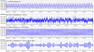Figure 8

Eq. (3) shows that, if a degree of fluid saturation of geological media and conductivity of the fluid do not change, then the process of transfer of charges in such media and changes of DEL charges' values on the borders of fluid and solid phases are under control of external electric field. For example, at an increase in intensity of external EMR, a density of conductivity current will grow, entailing reduction of density of DEL charges and will become the reason of activization of geoacoustic processes. It means that for seismically quiet periods changes of intensity of geoacoustic processes will be directly connected with changes of intensity of external electric field and conductivity of rocks around the borehole that explains the physical reasons of high correlation of RMS values of GAE and EMR signals on such time intervals. At the same time, it is necessary to emphasize that the connection of amplitudes of GAE responses with amplitudes of influencing EMR is of indirect character and manifests itself on relatively long intervals at measurement of RMS values of signals, isolation of an envelope, etc. Examples of manifestation of such effect are given in Figure 1 where it is shown that changes of RMS values of GAE registered in Boreholes G-1 and K-33, correspond to changes of RMS values of the external EMR registered in zones of the corresponding boreholes. In such sense it is possible to speak about an effect of modulating influence of EMR on the GAE level. On short (about 1 sec) time intervals the connection between intensity of external electric field and amplitude of GAE will not be so obvious. It is related to the fact that the GAE data reflect reorganization processes of geological media, occurring at the same time at different points of rather large volume of rocks of heterogeneous structure. Besides that, in various frequency bands the character of GAE variations can differ according to parameters of spectrum of influencing EMR. Figure 8 shows the examples of short (about 1 sec) realization of GAE and EMR signals received by results of synchronous geoacoustic and electromagnetic measurements in Borehole G-1.

Geoacoustic measurements were carried out at a depth of 1012 m. Beside the examples of realization of initial broadband (0.1–1000 Hz) GAE and EMR signals, Figure 8 shows the realization of signals of GAE and EMR for the frequency of 150 Hz, received by a narrow-band digital filtration of initial signals for the same time interval. Submitted data point out to the uncommon character of GAE responses to influence of external EMR that is caused by the reasons stated above. It is also possible to note that the comparison of structures and characters of changes in time of 150 Hz components for GAE and EMR channels (Figure 8c, Figure 8d). shows that in this case the geoacoustic component of 150 Hz is by no means the consequence of electromagnetic pickup. The data show that geoacoustic and electromagnetic components of the frequency of 150 Hz have different genesis, that can serve as a proof, first of all, of existence of deep amplitude modulation of the GAE component in the absence of that of the EMR component.

#### Increase of Compressive Stress Field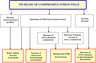Figure 9

The tendency of changes of parameters of geological media and responses of GAE to electromagnetic influence to the compressive stress increasing around the borehole can be presented in the form of a scheme in Figure 9. Increasing compressive stress can lead to a growth of the gradient of pore pressure [Sammonds et al., 1992], squeezing of fluid from zones of increased rock fracturing [Barsukov et al., 1985] and reduction of porosity of rocks.

An increase of a gradient of pore pressure and squeezing of fluid from zones of increased fracturing will lead to arrival of a part of fluid in borehole water owing to arrival to the water-bearing horizons of more mineralized steam solutions squeezed out from rocks, that will cause the corresponding increase in water mineralization of the borehole.

Squeezing of fluid from zones of increased fracturing under the influence of volume deformations will lead also to abrupt reduction of specific conductivity of geological media and to a corresponding significant increase in level of background electromagnetic radiation. Such conclusion is based on the research showing that even an insignificant reduction of fluid saturation of rock causes an abrupt reduction of specific conductivity [King and Smith, 1981]. According to Eq. (1) reduction of specific conductivity at squeezing of fluid from rock can entail reduction of conductivity currents and the corresponding reduction of GAE response to EMR influence. Owing to squeezing of fluid from rock the level of water in piezoemetric boreholes and outflow of self-streaming boreholes and sources can also increase.

Reduction of porosity together with the corresponding reduction of total area of free space in the volume of rock, contacting with the fluid and having DEL charges can cause reduction of total number of GAE sources, initiated by electro-kinetic processes and as a result – reduction of GAE level.

A further long increase of compressive tensions can lead to large-scale changes of capacity of pore/fracture space and conditions of filtration of underground waters [Kissin, 2009]. At this stage a mixture of waters of earlier isolated water-bearing horizons, strengthening or reduction of sub-current of deep fluids [Barsukov et al., 1985], and also synchronization of changes in water mineralization in boreholes located carried at considerable distances can occur. Responses of GAE to external electromagnetic influence at this stage can degrade almost completely.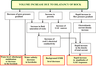Figure 10

At a change of a stage of growth of elastic deformations by a stage of dilatancy increase in rock volume [Nur, 1972] the increase of rock volume will lead to reduction of a gradient of pore pressure, increase of porosity of geological media and abrupt increase of a gradient of water current potential, and to a different characteristic scenario of evolution of parameters of fluid-saturated geological media (Figure 10).

The consequence of reduction of pore pressure gradient will be reduction of water mineralization of a borehole, stabilization or gradual reduction of water level (outflow) of the borehole. At that the level of fluid saturation of rock around the borehole can also increase, that would lead to an increase of thermal conductivity of rock that in its turn will reduce the level of background EMR.

Rapid increase of a gradient of water current potential [Eccles et al., 2005] will lead to significant growth of current of electro-kinetic origin. Together with increase of specific conductivity of geological media it will lead to an abrupt growth of density of the total current defining evolution of DEL charges and, respectively, to growth of amplitudes of GAE responses to EMR influence.

A consequence of increase of porosity of rock will be an increase of the total area of free surfaces in volume of rock contacting to the fluid and possessing DEL charges. It will cause an increase in total number of GAE sources initiated by electro-kinetic processes that will promote a growth of amplitudes of GAE response to electromagnetic influence.

Thus the process of dilatancy increase of rock volume will manifest itself in (see Figure 10):

1. reduction of water mineralization in boreholes;
2. stabilization and subsequent reduction (at a long period of dilatancy regime of deformation of geological media) of the level or water outflow from boreholes;
3. significant increase of amplitudes of GAE response to EMR in comparison to the GAE level, registered during the period of background seismicity.

It is obvious that the presented scenarios of evolution of parameters of fluid-saturated geological media at changes of its stress-strain state, are of general character and do not consider the features connected with characteristics of rocks around this or that point, configuration of a certain borehole, etc. Nevertheless, according to the results given below, this complex borehole measurements taken in the Petropavlovsk-Kamchatsky geodynamic polygon correlate rather borehole with the specified scenarios, and also with basic provisions of the dilatancy–diffusive (DD) model of preparation of earthquakes [Nikolayevsky, 1996; Scholz et al., 1973]. In accordance with the DD-model we have to take into account that the data of complex borehole measurements were obtained at a certain distance from the focal zones of earthquakes though in some cases the hypocentral distances for relatively strong ($M_{LH} \geq 6.0$) events didn't exceed 130 km. At the same time, according to the results of numerical modeling [Alekseev et al., 2001], at preparation of earthquakes a surface zone of dilatancy can develop which can significantly exceed horizontal sizes of an earthquake focal zone. Firstly, it explains existence of dilatancy volumes of geological media, and secondly, causes the relation of results of measurements in the Petropavlovsk-Kamchatsky geodynamic polygon with processes in zones of earthquakes' epicenters. Influence of processes of earthquake preparation on results of measurements can increase in cases of structural violations of solidity of geological media (active breaks of various scales, lineaments etc.) around a borehole, which at changes of a stress-strain state around their spreading zone can generate new zones of compaction and dilatancy [Goldin, 2002; Nikolayevsky, 1996].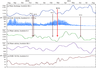Figure 11

In conclusion we would like to analyze the nature of change of a mode of rock deformation around Borehole G-1 in the time vicinity of the Tohoku megaearthquake including the data on the changes of level of background EMR (Figure 11). The data presented in Figure 11 indicate the significantly different characters of correlations between the series of measurements before and after the megaearthquake.

According to the data on changes of water outflow of Borehole GK-1 (Figure 11a), the level of compressive stress from the beginning of January and to the middle of February, 2011 was rather stable, and approximately a month before the megaearthquake there was a reduction of tension. During all specified interval a stable significant increase of amplitudes of GAE responses (Figure 11b). took place, the mineralization of water of Boreholes G-1 and GK-1 gradually decreased (Figure 11c, Figure 11d), the level of background EMR was low (Figure 11e). Such combination of parameters of changes of the influencing tension, GAE response, water mineralization of the boreholes and background EMR correlates with the variant of dilatancy increase of the rock volume (see Figure 10).

As it can be seen in Figure 11, approximately 12 days prior to the megaearthquake the level of background EMR started increasing rapidly and so did the water mineralization of GK-1. A bit later (approximately two days prior to the megaearthquake) the beginning of significant increase in mineralization of the top layer of water of Borehole G-1 (Figure 11c). was registered. On the basis of these data we can draw a conclusion that approximately two weeks before the megaearthquake, in the zone of the Petropavlovsk-Kamchatsky geodynamic polygon the active processes have begun, connected with squeezing of a fluid from rocks that can be interpreted as a transition of stress-strain state of geological environment to a stage of compaction.

The results of measurements taken on the interval of the middle of March–August, 2011 reliably correspond to the scenario of evolution of parameters of fluid-saturated geological media at increasing compressive stress around the borehole (see Figure 9). The increase of water outflow of Borehole GK-1 and of water mineralization of G-1 and GK-1 at simultaneous reduction of amplitudes of GAE response and the increase of level of background EMR correlate with this scenario. By the middle of April, 2011 the level of background EMR has grown approximately by 350% from the level of December, 2010 that testifies about abnormally high growth on this interval of specific resistance of geological media owing to intensive squeezing of a liquid fluid from zones of increased fracturing. The rapid reduction on this interval of amplitudes of GAE response up to its full degradation by July, 2011 confirms this conclusion.

### Conclusions

1. The nature of GAE response to external EMR in fluid-saturated geological media is defined by the values of density of the summed current, changing the values of DEL charges on the borders of fluid and solid phases. Generally a density of the summed current is a sum of three vector values – density of conductivity current, density of diffusive current and density of current of electro-kinetic nature. Regularities of changes of summed current are considerably connected with a stress-strain state of the geological media.
2. stabilization and subsequent reduction (at a long period of dilatancy regime of deformation of geological media) of the level or water outflow from boreholes;
3. significant increase of amplitudes of GAE response to EMR in comparison to the GAE level, registered during the period of background seismicity.
4. For seismically quiet periods changes of intensity of geoacoustic processes are directly connected with changes of intensity of external electric field and conductivity of rocks around a borehole. It explains the physical reasons of high correlation of RMS values of GAE and EMR on such time intervals. Conductivity of rocks thus acts as the parameter defining geoacoustic sensitivity of the geological media to influence of external electric field.
5. Effect of degradation of GAE response to the influence of EMR in time vicinity of relatively close strong earthquakes is related to decrease in conductivity and porosity of rocks around a borehole at a stage of compressive stress.
6. At a stage of dilatancy increase of the volume of rocks surrounding a borehole, a significant increase of amplitudes of GAE response to external electromagnetic influence takes place. The physical reasons of the specified effect are connected with abrupt increase of a gradient of capacity of the current, increase in porosity of the geological media, growth of fluid saturation and increase in specific conductivity of rock, characteristic for the specified stage of stress-strain state of the geological media.

#### Acknowledgments

The work was supported by the RFBR (grants 12-05-00670-a, 11-05-96005-r\_ural\_a), the Presidium of FEB RAS (grant 12-III-A-08-167) and the Program for the state support of leading scientific schools of the Russian Federation (grant NSh-5583.2012.5).

### References

Alexeev, A. S., A. S. Belonosov, V. E. Petrenko (2001), Concepts of multidisciplinary prediction of earthquakes using integral precursor, Problemy Dinamiki Litosfery i Seismichnosti. Vychislitelnaya Seismologia, 32, 81–97.

Asada, T., et al. (1984), Methods of Earthquakes Prediction. Their Use in Japan, Ed. by T. Asada, translated from English, Nedra, Moscow, 312.

Barsukov, V. L., G. M. Varshal, A. V. Garanin, N. S. Zamokhina (1985), Importance of hydrogeochemical methods for short-term prediction of earthquakes, Gidrogeohimicheskie Predvestniki Zemletryasenij, Nauka, Moscow, 3–16.

Bella, F., et al. (1998), Hydrogeochemical anomalies in Kamchatka (Russia), Phys. Chem. Earth, 921–925, doi:10.1016/S0079-1946(98)00120-7.

Belyakov, A. S. (2000), Magnetoelastic acoustic geophones for geophysical research, Seismicheskie Pribory, Issue 33, OIFZ RAN, Moscow, 27–45.

Biagi, P. F., A. Ermini, E. Cozzi, Y. M. Khatkevich, and E. I. Gordeev (2000), Hydrogeochemical precursors in Kamchatka (Russia) related to the strongest earthquakes in 1988–1997, Nat. Hazards, 263–276, doi:10.1023/A:1008178104003.

Biagi, P. F., L. Castellana, A. Minafra, G. Maggipinto, T. Maggipinto, A. Ermini, O. Molchanov, Y. M. Khatkevich, and E. I. Gordeev (2006), Groundwater chemical anomalies connected with the Kamchatka earthquake ($M=7.1$) on March 1992, Nat. Hazards Earth Syst. Sci., 853–859, doi:10.5194/nhess-6-853-2006.

Chelidze, T., et al. (2004), Electromagnetic initiation of slip: laboratory model, Acta Geofizika Polonica, 52, 1, 49–62.

Eccles, D., P. Sammonds, O. Clint (2005), Laboratory studies of electrical potential during rock failure, International Journal of Rock Mechanics, 42, 933–949, doi:10.1016/j.ijrmms.2005.05.018.

Finkel, V. M. (1977), Physical Basics of Deceleration of Destruction, Metallurgiya, Moscow, 359.

Fitterman, D. V. (1979), Theory of electrokinetic–magnetic anomalies in a faulted half-space, J. Geophys. Res., 84, 6031–6040, doi:10.1029/JB084iB11p06031.

Gavrilov, V. A. (2007), Physical reasons of daily variations of level of geoacoustic emission, DAN, 414, 3, 389–392.

Gavrilov, V. A. (2009), Dynamics of micro-fracturing of geological media in relation to the catastrophic Simushir earthquakes in 2006–2007 according to data of complex borehole measurements in the Kamchatka peninsula, Tectonic Physics and Actual Problems of Earth Sciences. The 40th anniversary of establishment by M. V. Gzovsky of the tectonic physical laboratory in IPE RAS. Materials of the All-Russia Conference, Vol. 2, IFZ, Moscow, 295–302.

Gavrilov, V. A., and Y. A. Vlasov (2011), Effect of modulation of level of geoacoustic emission by ultralow frequency radiation by results of measurements in different boreholes of the Petropavlovsk-Kamchatsky geodynamic polygon, Problems of Complex Geophysical Monitoring of the Russian Far East. Materials of the III Scientific Conference, 9–15 October 2011, Petropavlovsk-Kamchatsky, 207–211.

Gavrilov, V. A., Y. V. Morozova, and A. V. Storcheus (2006), Variations of level of geoacoustic emission in G-1 deep borehole (Kamchatka) and their relation to seismic activity, Vulkanologija i Seismologija, 1, 52–67.

Gavrilov, V., L. Bogomolov, Yu. Morozova, and A. Storcheus (2008), Variations in geoacoustic emissions in a deep borehole and its correlation with seismicity, Ann. Geophys., 51, 5/6, October/December 2008. 737–753.

Gavrilov, V. A., L. M. Bogomolov, and A. S. Zakupin (2011), Comparison of results of borehole geoacoustic measurements with laboratory data and full-scale experiments on electromagnetic influence on rocks, Fizika Zemli, 11, 63–74.

Goldin, S. V. (2002), Destruction of lithosphere and physical mesomechanics, Fizicheskaya Mezomekhanika, 5, 5, 5–22.

Goldin, S. V. (2004), Dilatancy, repacking and earthquakes, Fizika Zemli, 10, 37–54.

Khatkevich, Y. M., G. V. Ryabinin (2006), Hydrogeochemical research in Kamchatka related to search of earthquakes' precursors, Vulkanologija i Seismologija, 4, 34–42.

King, R. W. P., and G. S. Smith (1981), Antennas in Matters: Fundamentals, Theory and Applications, MIT Press, Cambridge, MA, 875.

Kissin, I. G. (2009), Fluids in the Earth crust: Geophysical and tectonic aspects, Nauka, Moscow, 328.

Kopylova, G. N., V. M. Sugrobov, and Y. M. Khatkevich (1994), Features of change of regime of sources and hydrogeological boreholes of the Petropavlovsk-Kamchatsky geodynamic polygon (Kamchatka) under the influence of earthquakes, Vulkanologija i Seismologija, 2, 53–70.

Krasjuk, N. P., and N. D. Dymovich (1974), Electrodynamics and Radio Waves Propagation, Vyshaya Shkola, Moscow, 536.

Nikolaevsky, V. N. (1996), Geomechanics and Fluid Dynamics, Nedra, Moscow, 447, doi:10.1007/978-94-015-8709-9.

Nur, A. (1972), Dilatancy, pore fluids and premonitory variations of $t_s/t_p$ travel times, Bull. Seism. Soc. Amer., 62, 5.

Rastogi, R. P., and R. C. Srivastava (1993), Non-equilibrium thermodynamics of electro-kinetic phenomena, Chem. Rev., 93, 6, 1945–1987, doi:10.1021/cr00022a001.

Report on results of drilling for search of thermal waters of Borehole G-1 (1988), "Khlebozavod", Site of Petropavlovskaya Square in 1986–1988, Petropavlovsk-Kamchatsky, 193.

Riznichenko, Y. V. (1976), Size of epicenter of crustal earthquake and seismic moment, Issledovaniya po Fizike Zemletryasenij, Nauka, Moscow, 9–26.

Ryabinin, G. V., and Y. M. Khatkevich (2009), Hydrogeochemical effects, preceding strong earthquakes in Kamchatka. Identification algorithm and morphological analysis, Vestnik KRAUNTS. Nauki o Zemle, Issue 13, 1, 107–122.

Ryabinin, G. V., Yu. S. Polyakov, V. A. Gavrilov, and S. F. Timashev (2011), Identification of earthquake precursors in the hydrogeochemical and geoacoustic data for the Kamchatka peninsula by flicker-noise spectroscopy, Nat. Hazards Earth Syst. Sci., 11, 541–548, doi:10.5194/nhess-11-541-2011.

Ryabinin, G. V., Yu. S. Polyakov, V. A. Gavrilov, and S. F. Timashev (2012), Cross-correlation earthquake precursors in the hydrogeochemical and geacoustic signals for the Kamchatka peninsula, Acta Geophysica, 60, 3, Jun. 2012, 874–893, doi:10.2478/s11600-012-0031-y.

Sammonds, P. R., P. G. Meredith, and I. G. Main (1992), Role of pore fluids in the generation of seismic precursors to shear fracture, Nature, 359, 228–230, doi:10.1038/359228a0.

Scholz, C. H., L. R. Sykes, and Y. P. Aggarval (1973), Earthquake prediction: A physical basis, Science, 181, 4102, 803.

Sidorin, A. Y. (1992), Precursors of Earthquakes, Nauka, Moscow, 192.

Svetov, B. S. (2002), Problem of frequency dispersion of conductivity of diphase geological media, Fizika Zemli, 1, 46–52.

Vlasov, Y. A., V. A. Gavrilov, V. P. Denisenko, and O. V. Fedoristov (2008), Telemetric system of complex geophysical monitoring network, Seismicheskie Pribory, Issue 44, OIFZ RAN, Moscow, 33–38.

Received 7 April 2013; accepted 17 April 2013; published 16 May 2013.Citation: Gavrilov V. A., I. A. Panteleev, G. V. Ryabinin, Yu. V. Morozova (2013), Modulating impact of electromagnetic radiation on geoacoustic emission of rocks, Russ. J. Earth Sci., 13, ES1002, doi:10.2205/2013ES000527.

Generated from LaTeX source by SemTeXML, v.1.2) software package.